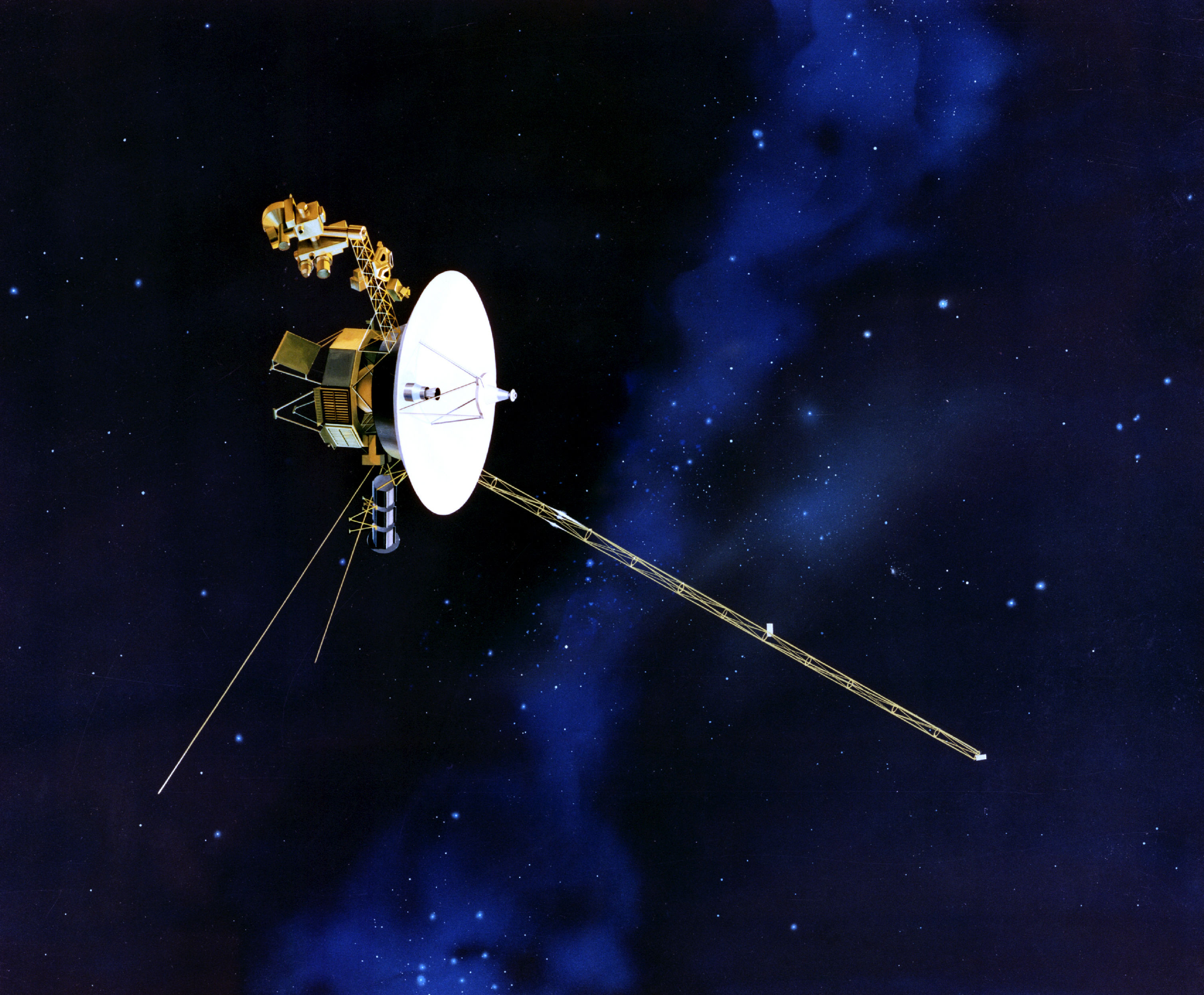## Why study exponents and their rules?Artist’s concept of Voyager in flight.

Mathematicians, scientists, and economists commonly encounter very large and very small numbers.  For example, Star Wars fans may remember Han Solo bragging about the Millennium Falcon’s ability to make the Kessel Run in less than 12 parsecs in Episode IV.  He was referring to a smuggler’s route with sections that were flown in hyperspace, making length an important factor in how quickly a ship could make the run.

In reality, a parsec is a unit of length used to measure large distances to objects outside the solar system. A parsec is equal to about $31$ trillion kilometers, or $19$ trillion miles in length. Rather than writing all the zeros associated with the number $1$ trillion $(1,000,000,000,000)$ we commonly use the written words or scientific notation, which is also called exponential notation. Scientific notation uses exponents to represent the number of zeros that come before or after the important digits of a very small or large number. Using scientific notation, $19$ trillion miles would be written ${1.9}\times{10}^{13}$ miles. In this example the number 13 is the exponent and the number 10 is referred to as the base.

The most distant space probe, Voyager 1, was $0.0006$ parsecs from Earth as of March 2015. It took Voyager $37$ years to cover that distance. Voyager 1 was launched by NASA on September $5, 1977$. As of $2013$, the probe was moving with a relative velocity to the sun of about $17030 \, m/s$. With the velocity the probe is currently maintaining, Voyager 1 is traveling about $325$ million miles per year, or $520$ million kilometers per year. Here are some more distances to well-known astronomical objects in parsecs:

• The distance to the open cluster Pleiades is $130$ parsecs from Earth. That’s ${1.7}\times{10}^{15}$ miles.
• The center of the Milky Way is more than $8$ kiloparsecs (a kiloparsec is $1000$ parsecs) from Earth, and the Milky Way is roughly $34$ kiloparsecs across.
• The nearest star to Earth (other than the sun), Proxima Centauri, is about $1.3$ parsecs from the sun.
• Most of the stars visible to the unaided eye in the nighttime sky are within $500$ parsecs of the sun.

In this section, you will learn the rules for algebraic operations in terms with exponents, then apply them to calculations involving very large or small numbers.

## Contribute!

Did you have an idea for improving this content? We’d love your input.